# How Many x-Intercepts?

## Question

What is the maximum number of zeros (also called x-intercepts) the function    may have? What is the minimum? c, a constant, may be any real number.

## Solution

The maximum number zeros of a polynomial function is equal to the function’s degree. The function  is fourth degree, so it may have up to four zeros. A fourth-degree function may look like this: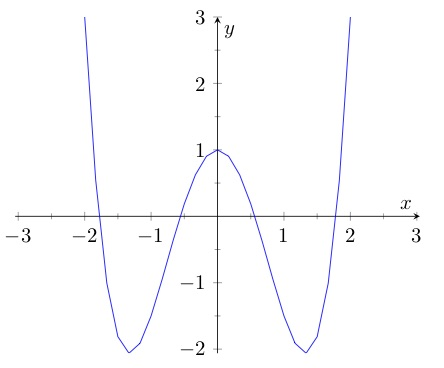Four is the max. Will the function  necessarily have four? Let’s try to narrow down the possibilities.

Try a graph. You can graph this function if you assign a value to c. Let’s try , so that the function is . That graphs up like this:That gives you two x-intercepts. but with a different value of c could this function may have more x-intercepts — or fewer?

At the x-intercepts, the y-value is 0:

That means

That means that for this function, at the zeros, the fourth-degree monomial   equals the linear function . Let’s graph these two pieces separately for  , the value of c that we’ve seen gives two x-intercepts.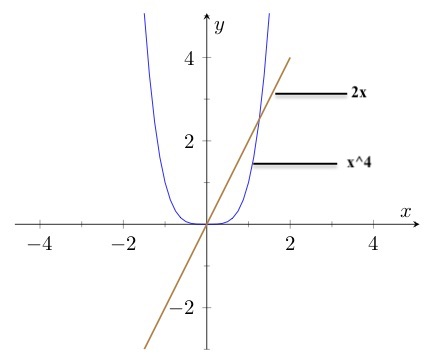These two pieces intersect in to placesone for each of the two zeros. Are any other points of intersection are possible? On the left-hand side, the fourth-degree term just keeps going up while the line just keeps going down, so there are no more points of intersection on the left. On the right-hand side to the right of both points of intersection, the fourth-degree term is always steeper than the line and is therefore always greater, so there are no more points of intersection on the right. Remember that each point of intersection on this graph corresponds to a zero on the function , so that means no other zero is possible for the function.

But that doesn’t cover all possibilities, because we considered only one value for c. What if c takes a different value? Then the line slides up or down. If  , the line slides up and there will two points of intersection, one at which x is less than zero and one in which x is greater than zero.

Slide it down just a little from and there are still two points of intersection, this time both positive. Slide it farther and eventually it will not intersect the fourth-degree curve at all.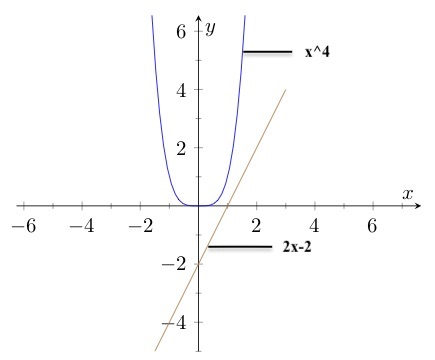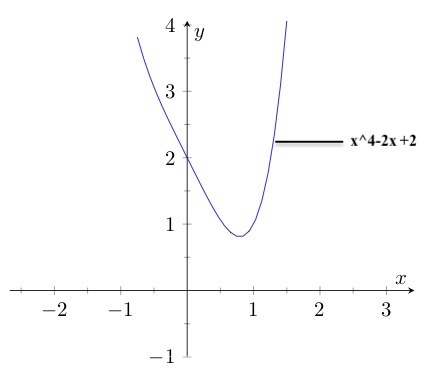For even higher values of c – meaning the line slides farther down on the y-axis – the function has no zeros.

So for the function , the minimum number of x-intercepts is zero and the maximum number is two.

# What’s up (more), the parabola or the line?

## Question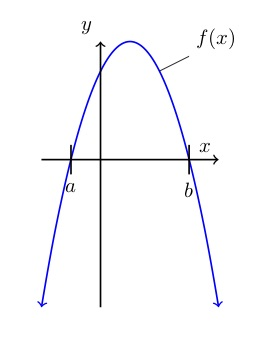The graph to the left shows the function f(x), a parabola – that is, a quadratic function. Not shown on the graph is function  in which c is a negative constant.

One statement below is true. Which one?

## Solution

What’s going on? The line will cross the parabola in a couple of places and those intersections will determine which function is higher in various places. But all you know about function is that  and that c is negative.

is the equation of a straight line. Think of a line’s basic equation:  where b is the line’s y-intercept – that is, y’s value where . In this case, because  nothing, the y-intercept is 0. That means the line goes through the origin.

Also, you’re told that c is negative. c is in the role of m in the equation that is, it’s the slope. The negative slope means this line goes down as it goes to the right.

How steep is the line? You are given no information about that. Take a guess.

The brown line in the graph to the right shows what function g may look like. It may be steeper or less steep than shown,but it will cross the x-axis at the origin, and it will go down as it goes to the right, as shown.

Let’s go through all the possible answers to find the one true statement.

1. . At , as you can see from the graph, the parabola is above the x-axis, which means f(0) is a positive value. The line goes right through the origin – so . Thus which means it cannot be true that .
2. . At , function f crosses the x-axis. So . At , function g crosses the x-axis. So . That means f(a) is equal to g(0), so it’s not possible that f(a) is less than g(0).
3. . What’s happening at ? Function f crosses the x-axis there, so ; and function g has a positive value. That means g is more than f, so it must be that . Looks like that’s the answer, but let’s still check the others.
4. . We have established that . And since g(x) goes through the origin and slopes down, g must be less than zero at any x-value that is greater than 0 – like, for example, b. That means g is less than f, so it is not possible that f is less than g.
5. . As you can see from the graph, . And, as we said in response to answer #4, g(b) is less than 0. So f(b) must be more than g(b) – and it’s not possible that .